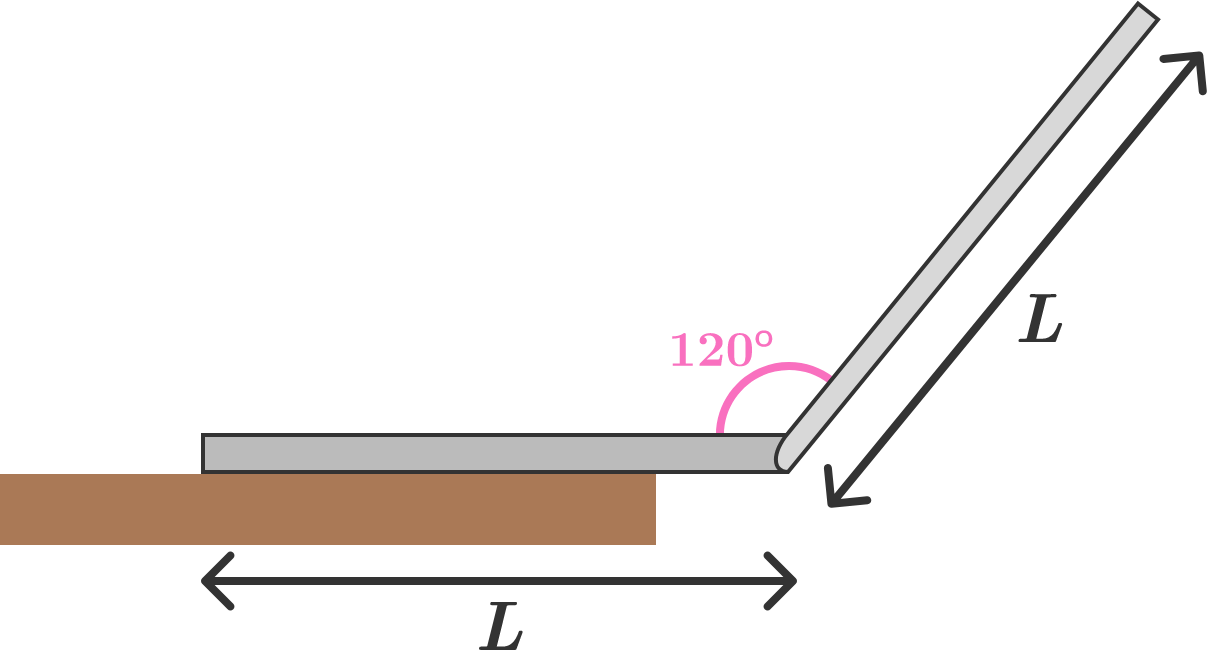# Precariously Perched LaptopA student is preparing feverishly for final exams, and he doesn't realize that his laptop has been creeping towards the edge of his desk and is about to fall off.

What fraction of the base of the laptop can hang off of the edge of the desk before the entire thing falls off?

Details and Assumptions:
$\hspace{0.5cm}\bullet$ The base of the laptop and the top (screen) portion both have the same length $L$.
$\hspace{0.5cm}\bullet$ Both the base and the screen have uniform mass density as a function of length.
$\hspace{0.5cm}\bullet$ The base of the laptop is twice as massive as the top (screen) portion.
$\hspace{0.5cm}\bullet$ The screen makes an angle of 120 degrees with respect to the base.
$\hspace{0.5cm}\bullet$ Neglect the thickness of the base and of the screen.
$\hspace{0.5cm}\bullet$ The 120-degree angle mentioned above is the only non-right angle in this problem.
$\hspace{0.5cm}\bullet$ Treat the laptop, as a whole, as a rigid body.
$\hspace{0.5cm}\bullet$ Give your answer as a decimal number between 0 and 1.

×